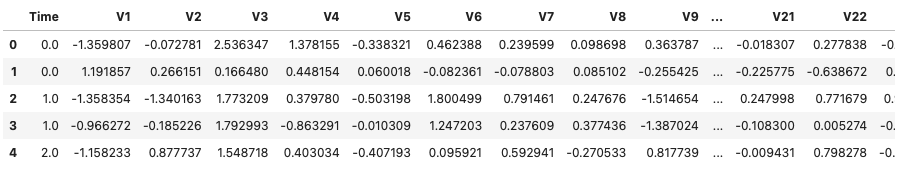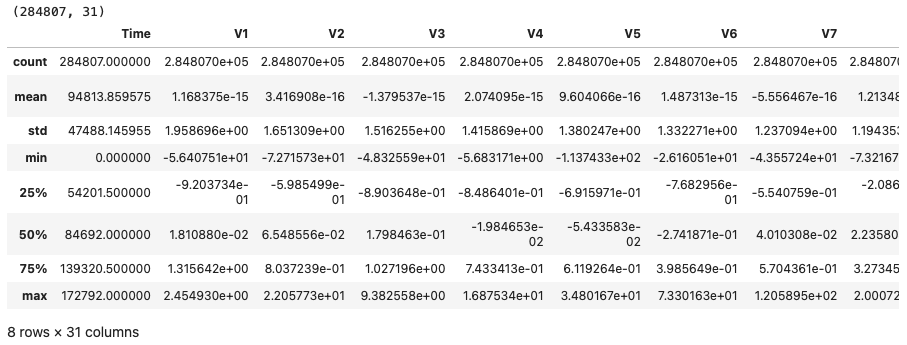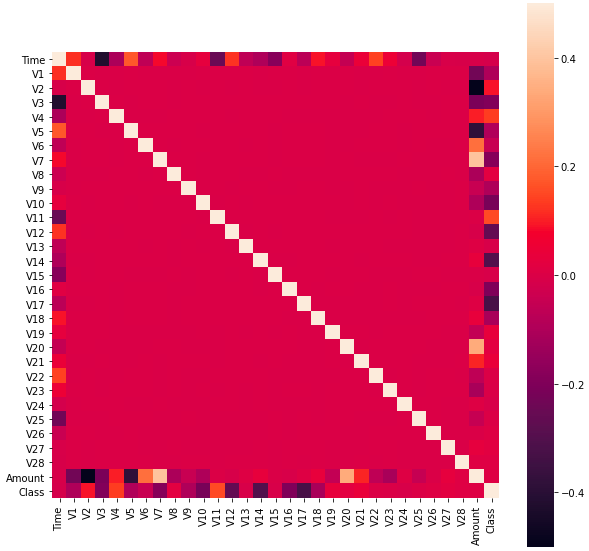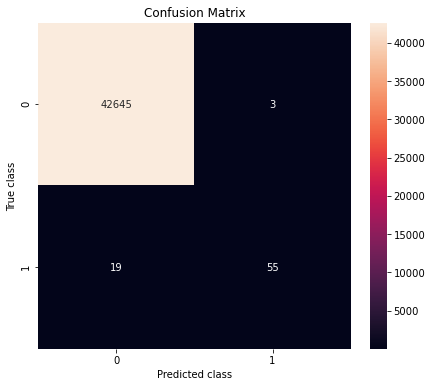# Credit Card Fraud Detection using Random Forests

## Introduction

Before proceeding towards the model, let us understand what credit card fraud is and how it happens. Credit card fraud is an unauthorized transaction done without your credit card. Thieves access the credit card credentials by phishing or credit card skimming. So by detecting such fraud, customers will not be charged for the items they did not purchase.

## Challenges Involved

• A lot of transactions are processed throughout the day, so an enormous amount of data will be generated. Our model must be fast enough to detect the fraud within no time.
• The number of fraud transactions throughout the day will be less, so the data will be imbalanced.
• Availability of the data is very difficult because most of the transactions are private.
• Incorrect classification of the data is another major issue, as every fraud transaction is not reported so they will be marked as valid transactions.
• The scammers use robust methods against the models.

## Tackling the challenges

• The model must be simple and fast so that fraudulent transactions are identified in less span of time.
• Imbalance datasets can be handled using many techniques like downsampling and upsampling.
• We can apply principal component analysis to the data to maintain the user's privacy.
• It is necessary to have a trustworthy source for the data extraction in order to get a better model.
• Make a simple and interpretable model so that when the scammer gets adapted to it, you can immediately change the model with some tweaks.

So let's start the implementation of the model. Before implementing the model download the data from this link. We will be making our model in the jupyter notebook.

## Implementation

Importing all the necessary libraries.

``````import pandas as pd
import numpy as np
import seaborn as sns
import matplotlib.pyplot as plt
from matplotlib import gridspec``````

Load the downloaded dataset using pandas. A correct path should be provided in order to load the dataset properly. It's better to keep the dataset and the model in the same directory.

``````# loading the dataset

Let us analyze the data.

``data.head()``Here Time, V1, V2, … are independent features of the dataset. There is no name, card number, or any other information in the dataset because the original was formatted using principal component analysis in order to protect the user's privacy.

``````# getting shape and other details of the data
print(data.shape)
data.describe()``````Our dataset has 284807 data points and 31 columns. In 31 columns. there are 30 independent features and 1 dependent feature.

Now, let us check how imbalanced our dataset is.

``````fraud_ = data[data["Class"] == 1]
valid_ = data[data["Class"] == 0]
print("Total fraud transactions: ",len(fraud_))
print("Total valid transactions: ",len(valid_))``````
``````Total fraud transactions:  492
Total valid transactions:  284315``````

As we can see that there are only 492 fraudulent transactions and 284315 valid transactions. It means that the dataset is highly imbalanced. Not even one percent of the data has fraud transactions. So it indicates that the dataset is highly imbalanced. (A perfectly balanced dataset has an equal number of observations for all possible level combinations)

Let us train a model using this unbalanced dataset. If the results are not good, we can change the imbalanced dataset to a balanced one by downsampling or upsampling the dataset.

Checking the amount details of fraudulent and valid transactions.

``fraud_.Amount.describe()``
``````count     492.000000
mean      122.211321
std       256.683288
min         0.000000
25%         1.000000
50%         9.250000
75%       105.890000
max      2125.870000
Name: Amount, dtype: float64``````
``valid_.Amount.describe()``
``````count    284315.000000
mean         88.291022
std         250.105092
min           0.000000
25%           5.650000
50%          22.000000
75%          77.050000
max       25691.160000
Name: Amount, dtype: float64``````

Observe that the average amount of fraudulent transactions is more than that of valid ones. Due to this, it is necessary to predict whether a transaction is fraudulent or valid.

Plotting a correlation matrix. The correlation matrix gives us an idea about how features can correlate with each other. They highlight the features that are necessary for the prediction.

Correlation: If the value of correlation is positive then the two variables move with coordination in the same direction, and if the value is negative then two variables move with coordination in the opposite direction. The value of the correlation coefficient lies between -1 to 1. 1 indicates that two variables have a perfect positive relationship and -1 indicates that the two variables have a perfect negative relationship.

``````corrMatrix = data.corr()
fig = plt.figure(figsize=(10,10))
sns.heatmap(corrMatrix, square = True, vmax = .5, vmin = -.5)
plt.show()``````From the following heatmap, we can say that many of the features do not correlate which each other. V7 and V20 are features that are positively related to the Amount feature. At the same time, V2 and V5 are the features that are negatively related to the Amount feature.

Now separate the dependent and independent features of the dataset for training, i.e., separate the data into X and Y where X is input data and Y is output data.

``````y = data["Class"]
x = data.drop(["Class"], axis = 1)
print(x.shape, y.shape)
# converting it to numpy array with no column
Ydata = y.values
Xdata = x.values``````
``(284807, 30) (284807,)``

Splitting the dataset for training and testing using sklearn’s train_test_split.

``````# importing train_test_split
from sklearn.model_selection import train_test_split
xtrain, xtest, ytrain, ytest = train_test_split(Xdata, Ydata, random_state = 42, test_size = 0.15)``````

Build a model by random forest classifier algorithm. We will import a random forest classifier from the sklearn library.

``````# importing rfc classifier
from sklearn.ensemble import RandomForestClassifier
RFC = RandomForestClassifier()
RFC.fit(xtrain, ytrain)
ypred = RFC.predict(xtest)``````

Summary of the model.

``````# calculating every score of the model
from sklearn.metrics import classification_report, accuracy_score, precision_score, recall_score, f1_score, matthews_corrcoef, confusion_matrix

n_outliers = len(fraud_)
n_errors = (ypred != ytest).sum()
print("Random Forest Classifier")

# calculating accuray of the model
accuracy = accuracy_score(ytest, ypred)
print("Accuracy : ",accuracy)

# calculating precision of the model
precision = precision_score(ytest, ypred)
print("Precision: ",precision)

# calculating recall score of the model
recall = recall_score(ytest, ypred)
print("Recall: ",recall)

# calculating f1-score of the model
f1 = f1_score(ytest, ypred)
print("F1-Score: ",f1)

# calculating matthews correlation coefficient of the model
MCC = matthews_corrcoef(ytest, ypred)
print("Matthews correlation coefficient: ",MCC)``````
``````Random Forest Classifier
Accuracy :  0.9994850428350732
Precision:  0.9482758620689655
Recall:  0.7432432432432432
F1-Score:  0.8333333333333333
Matthews correlation coefficient:  0.839286576513758``````

Visualizing the confusion matrix for the above predictions.

``````# printing the confusion matrix
conf_matrix = confusion_matrix(ytest, ypred)
plt.figure(figsize = (7, 6))
sns.heatmap(conf_matrix, annot=True, fmt ="d");
plt.title("Confusion Matrix")
plt.ylabel('True class')
plt.xlabel('Predicted class')
plt.show()``````## FAQs

1. What is the random forest with an example?

A: Random forest is an algorithm consisting of many decision trees. Suppose there are 4 trees in a random forest. Three of them predicted that the transaction was fraudulent, and only one predicted that the transaction was valid. As most decision trees have said transaction is fraudulent, the random forest will give the output as fraudulent.

2. When should we random forest?

A: Random Forest is suitable for situations when we have a large dataset, and interpretability is not a major concern. Decision trees are much easier to interpret and understand. It becomes more difficult to interpret since a random forest combines multiple decision trees.

3. Why is the random forest algorithm so good?

A: Random forests are great with high-dimensional data since we work with subsets of data. It is faster to train than decision trees because we work only on a subset of features in this model, so we can easily work with hundreds of features.

4. Does random forest reduce overfitting?

A: Random Forests do not overfit. The testing performance of Random Forests does not decrease (due to overfitting) as the number of trees increases. Hence after a certain number of trees, the performance stays at a certain value.

## Key Takeaways